# “The laws of nature are but the mathematical thoughts of God.” — Euclid

The ancients claimed that God works by mathematics. Their religion was a conglomeration of religion, astrology, alchemy, physical and mental science, and mathematics. Ancient astrology divided the starry heavens into 36 constellations. These were represented by different amulets called “Sigilla Solis,” or the sun seal. These amulets were worn by the pagan priests, and they contained all the numbers from 1 to 36. “

— Roy Allan Anderson, “Unfolding the Revelation”

## God Works by Numbers

Science, and particularly geometry and astronomy, were linked directly to the divine for ancient builders of temples and monuments. Since God created the universe after geometric and harmonic principles, to seek these principles was therefore to seek and worship God.God as Architect/Builder/Geometer/Craftsman, Codex Vindobonensis 2554 (French, ca. 1250),
in the Österreichische Nationalbibliothek., The Frontispiece of Bible Moralisee

## 666 and the Magickal Seal of the Sun

The “Seals of the Planets,” popular before the time of Christ according to Budge (Amulets and Superstitions), are interesting because the seal containing “the Grand Number of the Sun” contains the very sacred number 36 laid out in a 6×6 square with the numbers from 1 to 36 so arranged that they add up the same in all directions, with the total of the whole seal 666.

Though popular also in Eastern lands, the Greek and Roman, or Latin, inscriptions on these seals show also their popularity in the West.. Since the sun-god was considered as the ruler over the 36 constellations of the sky and the 36 rooms of the circle of the zodiac, it was inevitable that the summary number of the numbers from 1 to 36, the number 666 should have been assigned to the sun as the ruler over all the gods of heaven and earth.

Babylonian astrologers divided the starry heavens into 36 constellations (ten days each). These were represented by different amulets called “Sigilla Solis,” or the Sun Seal. These amulets were worn by the pagan priests and they contained all the numbers from 1 to 36. By these figures they claimed to be able to foretell future events. These amulets were usually made of gold, yellow being the sun color. While being carried, these amulets were wrapped in yellow silk, as it was thought that the bearer would thus receive the beneficent powers believed to emanate from that jewel.

Adding the numbers of any column either horizontally or vertically, and also the two diagonals crossing the square, the total is the same — 111. The sum of the six columns, either horizontally or vertically, is 666. The illustrations below taken from actual amulets in the Berlin Museum.The 6×6 Magic Square of the Sun contains the first 36 numbers arranged in such a fashion so that each line of numbers, whether added horizontally, vertically or diagonally from corner to corner, will yield the “solar number” 111. The entire magic square therefore equals 666, a number which was significant to early Christian mystics.   In Hebrew Kabbalah, the names of the intelligence of the Sun and Spirit of the Sun were designed to equal 111 and 666 respectively. Like 888, 666 is an important musical number, for .666 is the ration of the perfect fifth, the most powerful harmonic interval.  — From Jesus Christ, Sun of God (Fidler)“The second illustration is also a solar seal, but it honors the star Basilisco, which was the diminutive form of the Greek basileus (king), thus meaning the same as the Latin “regulus”. Now, Regulus is the only first-magnitude star in the constellation of Leo. The sun and the moon are again clearly seen on this amulet, and on the reverse side is the same arrangement of numerals, with the actual figure given of the total 666.”

The lower coin shows the conjunction of the Sun, Moon and the star Regulus in the constellation of Leo the Lion that occurred on 19 August 1705.

Table #1 as illustrated above is also referred to in numerology as the magic Square of the Sun. There are similar magic squares for Saturn, Jupiter, Mars, Venus, Mercury and the Moon, all of which have been known for centuries.

So 666 is a number associated with pagan sun-worship, which originated in the mysteries of ancient pagan Babylon. In Revelation 666 is associated with the number of the beast (from the sea), the Antichrist, and the harlot church of Mystery Babylon.

This ‘magic square of the sun’ is associated with the ‘The Philosophers stone’:The Philosopher’s stone is associated with the hexagram…a symbol that comes up over and over again in connection with the number 666.

The Hexagram of Earth represents the Solar energy that makes concrete matter that we can move and touch, in short, The Philosophers Stone.

Dr. John Dee, the court astrologist of Queen Elizabeth I, in his book Hieroglyphic Monad, includes the following quote:

“‘Mahatma Letters,’ page 345: ‘The double triangle viewed by the Jewish Kabbalists as Solomon’s Seal is…the Sri–Antana of the Archaic Aryan Temple, the Mystery of Mysteries, a geometrical synthesis of the whole occult doctrine. The two interlaced triangles are the Buddham-Gums of Creation. They contain the ‘squaring of the Circle,’ the ‘Philosophers’ Stone,’ the great problems of Life and Death–the mystery of Evil.The Chela who can explain this sign from every one of its aspects is virtually an Adept.’”John Dee, Hieroglyphic Monad, Dr John Dee, WEISER BOOKS, Boston MA/York Beach, ME, page 76

This portion taken R. Allan Anderson’s “Unfolding the Revelation”:

The Babylonians, who invented the zodiac, considered each of the twelve signs to rule over the twelve months of the year. They further divided each of the 12 houses into 3 rooms. 12 x 3 = 36.

Each of the 36 designated gods ruled over his own 10 degrees of the 360 zodiac and over his 10 days of the 360-day zodiacal year. It was believed that no god would strike you if you were wearing his identifying amulet. It would thus behoove you to invoke the numbers of each of the gods. The number which encompasses them all is 666. An amulet which contained all of these numbers would insure the favor of all the gods, anywhere, anytime.  But the ancients didn’t stop with using magic numbers in serial order. Arranging these numbers in a magic square could greatly compound their power.Each line, column or diagonal adds up to 111. The sum of the six lines or six columns is 666. With the numbers arranged thus in magic squares, you can hope to invoke the full power of the sun, the king of the gods. His number is 666. (See bottom for coins and illustrations from the book.)

Just as to the ancients, the number 7 signified completeness or perfection, the number 6 could represent something less than completion, or a contrast to perfection. Consider our term “hex,” with its connotations of evil, magic and witchcraft. Yet the word is merely the number 6.

Quite obviously, the “666” has existed in religions since ancient antiquity. So we must then inquire as to how this came to mean so much.  In ancient religions, Gods had a triple aspect to them, which was the creator, the maintainer, and the destroyer.

## Sacred Tetractys

The Pythagoreans adored numbers. Aristotle, in his Metaphysica, sums up the Pythagorean’s attitude towards numbers.

“The (Pythagoreans were) … the first to take up mathematics … (and) thought its principles were the principles of all things. Since, of these principles, numbers … are the first, … in numbers they seemed to see many resemblances to things that exist … more than [just] air, fire and earth and water, (but things such as) justice, soul, reason, opportunity”One of the fascinating ancient discoveries is Tetractys. It is a symbol composed of ten dots in an upward-pointing triangular formation. It was a sacred pattern for the school of philosophers who followed the teachings of the Greek sage Pythagoras.

Tetractys itself can be interpreted as the symbolic blueprint of creation. Its image is an equilateral triangle based on the essential numbers 1 (top), 2, 3 and 4 (base), whose sum is the “perfect” number 10  ( 1 + 2 + 3 + 4 = 10).

These numbers were considered by the Pythagoreans to be holy and at the origins of the universe. They believed that a four-fold pattern permeated the natural world, examples of which are the  point, line, surface and solid and the four elements Earth, Water, Air and Fire.

Musically they represent the perfect consonants: the unison, the octave, the fifth and the fourth.

The importance of the tetractys to the Pythagoreans is illustrated by their oath of fellowship:

I swear by the discoverer of the Tetractys,
Which is the spring of all our wisdom,
The perennial fount and root of Nature.Tetractys generates hexagon which is 2-D projection of 3-D cube

Sacred Tetractys

One particular triangular number that they especially liked was the number ten. It was called a Tetractys, meaning a set of four things, a word attributed to the Greek Mathematician and astronomer Theon (c. 100 CE). The Pythagoreans identified ten such sets.

In Plato’s Timaeus, we find that God created the Cosmic Soul using two mathematical strips of 1, 2, 4, 8 and 1, 3, 9, 27. These two strips have the shape of an inverted “V” or the “Platonic Lambda” since it resembles the shape of the 11th letter of the Greek alphabet “Lambda” (?).The Platonic Lambda interestingly describes 3 dimensional space:

• Top – 1 — single point
• 1st  row: 2 – 3 — linear dimension
• 2nd  row: 4 – 9  — surface (2-D: 2×2 and 3×3)
• 3rd  row: 8 – 27 — cubic volume (3-D: 2x2x2  and 3x3x3)

## The Summation Series (Fibonacci)

The natural progression follows a “summation series” that is known today as the Fibonacci Series [ of course this Series was in existence before Fibonacci (born in 1179 CE) – he simply “re-discovered” it.] The Summation Series is a progressive series, where you start with the first two numbers, then you add their total to generate the next number, and so on. By definition, the first two Fibonacci numbers are 0 and 1, and each subsequent number is the sum of the previous two. The first summation progression:

1, 2, 3, 5, 8, 13, 21, 34, 55, 89, 144, 233, 377, …

It is worth to note that the first summation (Fibonacci) progression generates  approximation of the golden ratio (PHI):

3/2 =1.5000
5/3 =1.666…
8/5 =1.6000
13/8 =1.6250
21/13=1.6154…
34/21=1.6190…
55/34=1.6176…
89/55=1.61818…
144/89=1.618….

The second of Fibonacci progression (provides approximate geometry of the pentagram and also lead to golden ratio ‘phi’ just like in the above example:  322/199=1.618… ):

1, 3, 4, 7, 11, 18, 29, 47, 76, 123, 199, 322, …

The Summation Series is reflected throughout nature. The number of seeds in a sunflower, the petals of any flower, the arrangement of pine cones, the growth of a nautilus shell, etc…all follow the same pattern of these series.

The overwhelming evidence indicates that the Summation Series was known to the Ancient Egyptians. Throughout the history of Ancient Egypt, temples and tombs (including pyramids) show in their design  expression of the Summation Series: 2, 3, 5, 8, 13, 21, 34, 55, 89, 144, 233, 377, 610, . . .

Read More (our previous blog entry): http://blog.world-mysteries.com/science/sacred-geometry

## Polygonal Numbers

A figurate number, also known as a figural number, is a number that can be represented by a regular geometrical arrangement of equally spaced points. If the arrangement forms a regular polygon, the number is called a polygonal number. The polygonal numbers illustrated above are called triangular, square, pentagonal, and hexagonal numbers, respectively.

### Polygonal Numbers:### The Triangular Numbers:The triangular number Tn is a “figurate number” (a number that can be represented by a regular geometrical arrangement of equally spaced points) that can be represented in the form of a triangular grid of points where the first row contains a single element and each subsequent row contains one more element than the previous one. This is illustrated above for T1=1, T2=3, …. The triangular numbers are therefore 1, 1+2, 1+2+3, 1+2+3+4, …, so for n=1, 2, …, the first few are 1, 3, 6, 10, 15, 21, …

### Flower of Life and Figural Numbers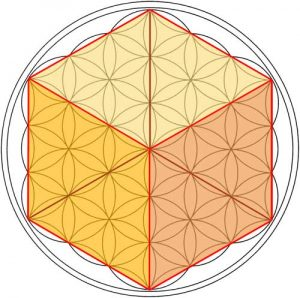2-D projection of the Cube can be found in the Flower of Life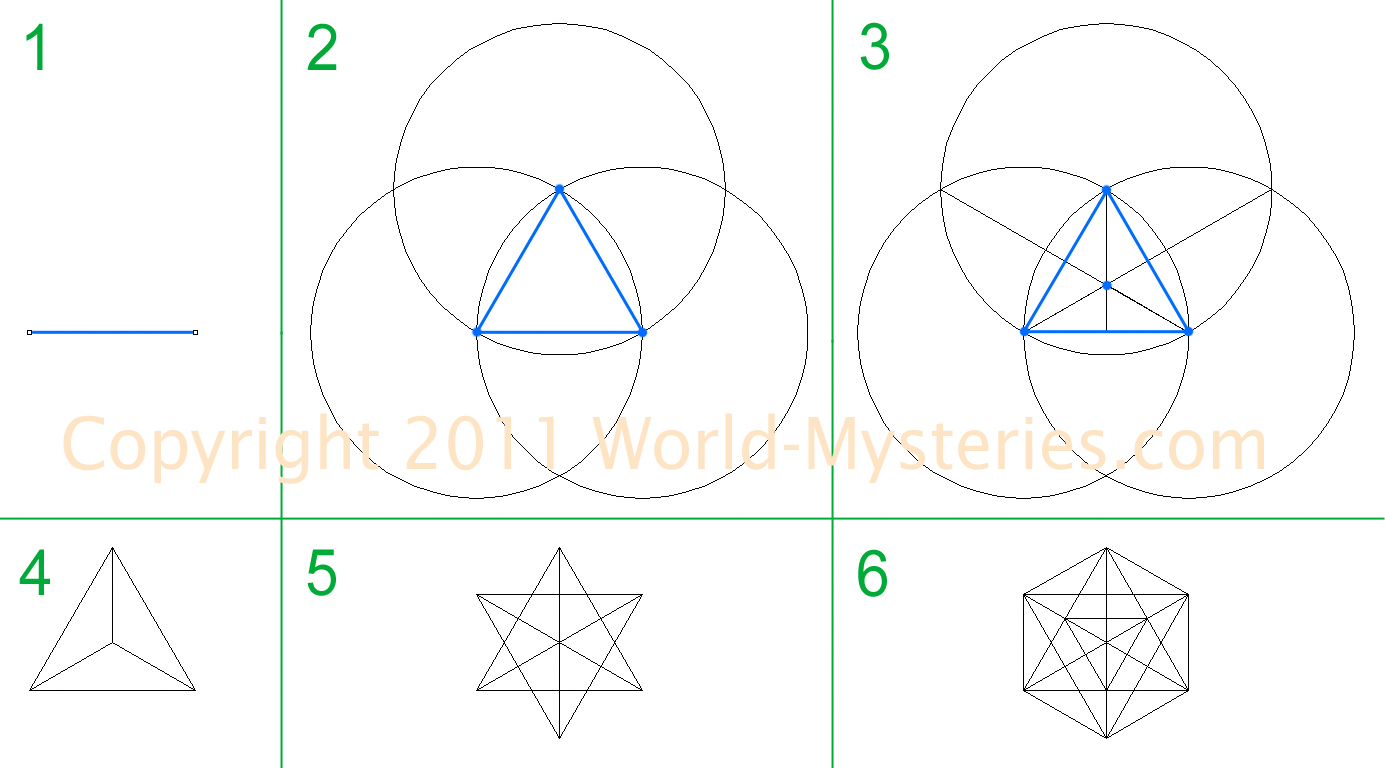Simple method of creating 2-D projection of Platonic solids.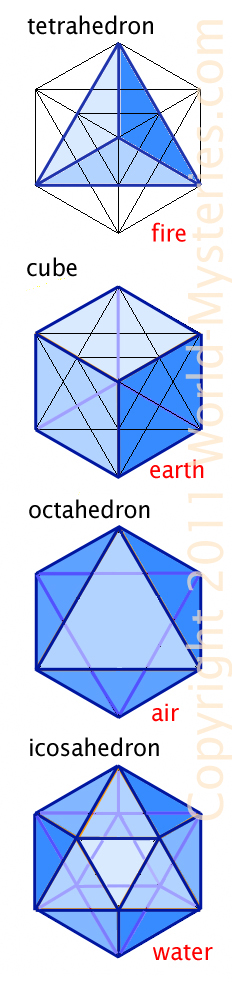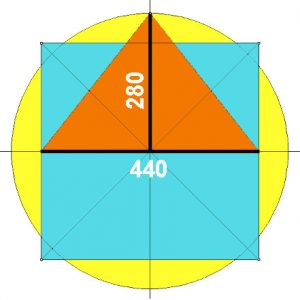Principle of the Great Pyramid design (image above)
based on the “squared circle” with “Pi” as 22/7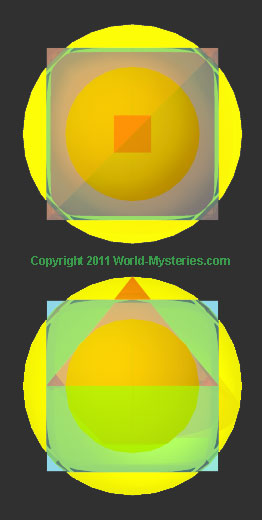Top and front view of the 3D image of the GP
based on the “squared circle”.
Cube’s edge becomes the base side of  the GP
and radius of the sphere becomes it’s height
(44o x 280 Royal Cubits).

Below: 2 perspective views of the above design: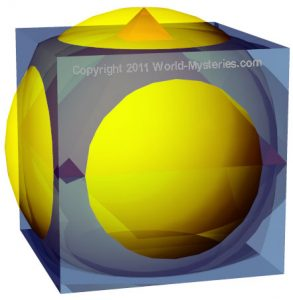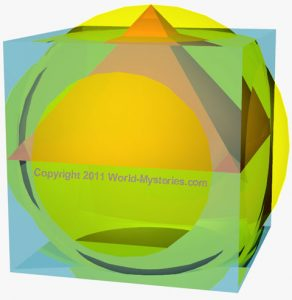## Patterns in Numbers and Pascal’s Triangle

In mathematics, Pascal’s triangle is a triangular array of the binomial coefficients in a triangle. It is named after the French mathematician, Blaise Pascal. It is known as Pascal’s triangle in much of the Western world, although other mathematicians studied it centuries before him in India, Persia, China, Germany, and Italy.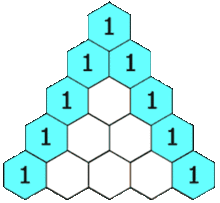The rows of Pascal’s triangle are conventionally enumerated starting with row n = 0 at the top. The entries in each row are numbered from the left beginning with k = 0 and are usually staggered relative to the numbers in the adjacent rows. A simple construction of the triangle proceeds in the following manner. On row 0, write only the number 1. Then, to construct the elements of following rows, add the number directly above and to the left with the number directly above and to the right to find the new value.If either the number to the right or left is not present, substitute a zero in its place. For example, the first number in the first row is 0 + 1 = 1, whereas the numbers 1 and 3 in the third row are added to produce the number 4 in the fourth row.

The set of numbers that form Pascal’s triangle were well known before Pascal. However, Pascal developed many applications of it and was the first one to organize all the information together in his treatise, Traité du triangle arithmétique (1653).

The numbers originally arose from Hindu studies of combinatorics and binomial numbers and the Greeks’ study of figurate numbers.

The earliest explicit depictions of a triangle of binomial coefficients occur in the 10th century in commentaries on the Chandas Shastra, an Ancient Indian book on Sanskrit prosody written by Pingala in or before the 2nd century BC. While Pingala’s work only survives in fragments, the commentator Halayudha, around 975, used the triangle to explain obscure references to Meru-prastaara, the “Staircase of Mount Meru”. It was also realised that the shallow diagonals of the triangle sum to the Fibonacci numbers. In 1068, four columns of the first sixteen rows were given by the mathematician Bhattotpala, who realized the combinatorial significance.

At around the same time, it was discussed in Persia (Iran) by the Persian mathematician, Al-Karaji (953–1029). It was later repeated by the Persian poet-astronomer-mathematician Omar Khayyám (1048–1131); thus the triangle is referred to as the Khayyam triangle in Iran. Several theorems related to the triangle were known, including the binomial theorem. Khayyam used a method of finding nth roots based on the binomial expansion, and therefore on the binomial coefficients.

In 13th century, Yang Hui (1238–1298) presented the arithmetic triangle that is the same as Pascal’s triangle. Pascal’s triangle is called Yang Hui’s triangle in China. The “Yang Hui’s triangle” was known in China in the early 11th century by the Chinese mathematician Jia Xian (1010-1070).

Petrus Apianus (1495–1552) published the triangle on the frontispiece of his book on business calculations in the 16th century. This is the first record of the triangle in Europe.

In Italy, it is referred to as Tartaglia’s triangle, named for the Italian algebraist Niccolò Fontana Tartaglia (1500–77). Tartaglia is credited with the general formula for solving cubic polynomials, (which may in fact be from Scipione del Ferro but was published by Gerolamo Cardano 1545).

Pascal’s Traité du triangle arithmétique (Treatise on Arithmetical Triangle) was published posthumously in 1665.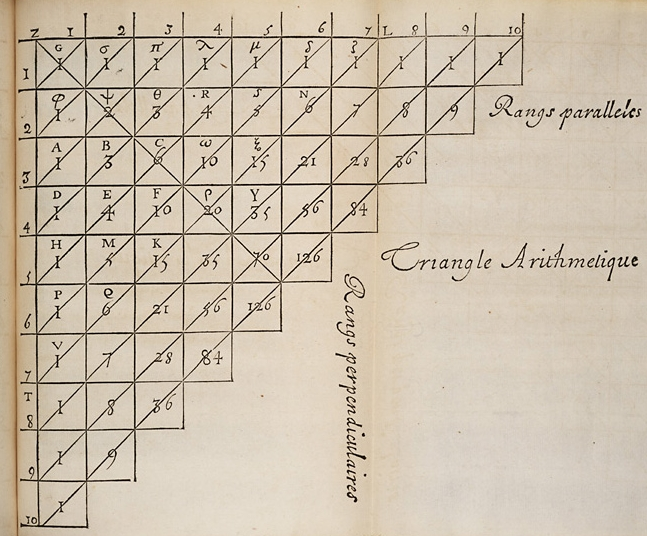Blaise Pascal’s version of the triangle.

Pascal’s triangle has many amazing properties and contains many “special” patterns of numbers. Below we are presenting few of the most incredible examples.Odd and Even numbers form the Sierpinski Triangle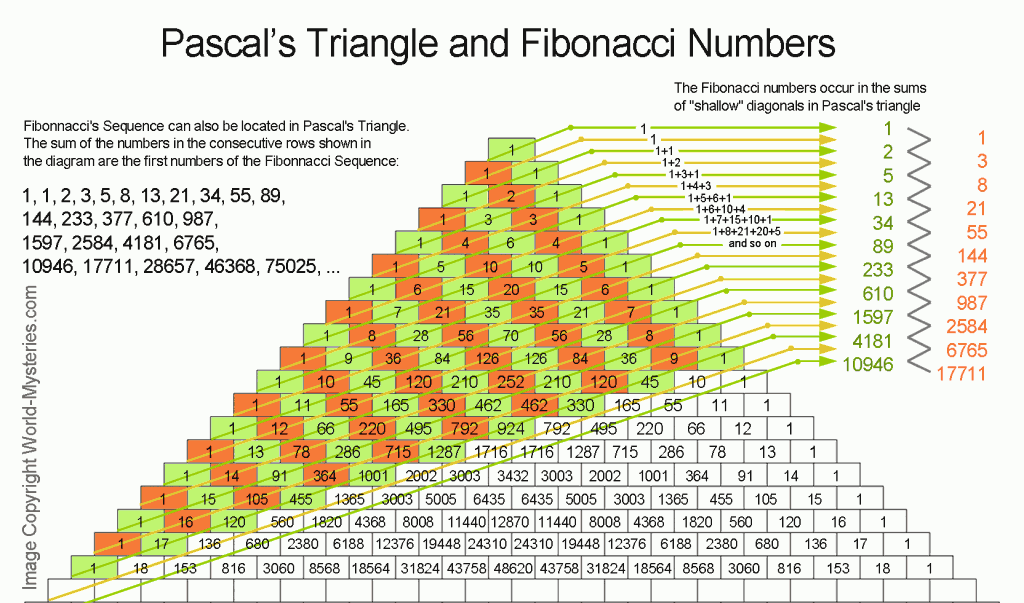Fibonacci Numbers are generated by the Pascal’s Triangle.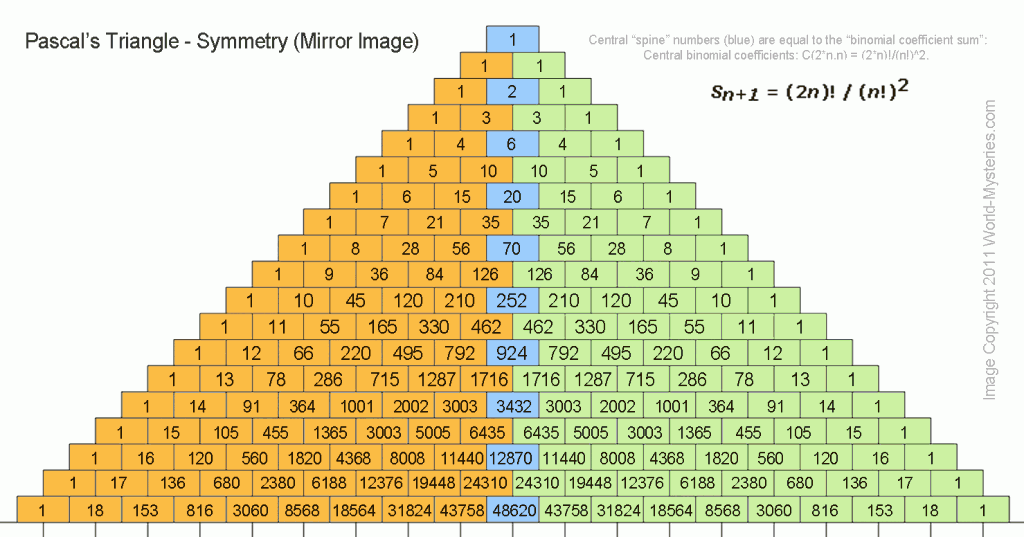Number’s Symmetry and the”binomial coefficient expansion” numbers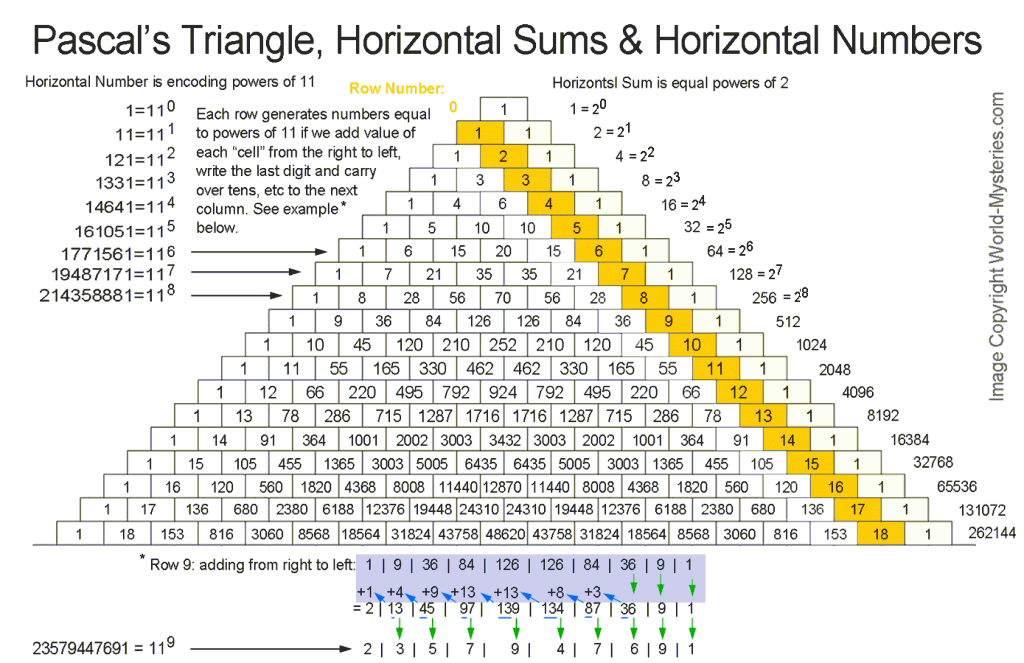Powers of 2 and 11 series.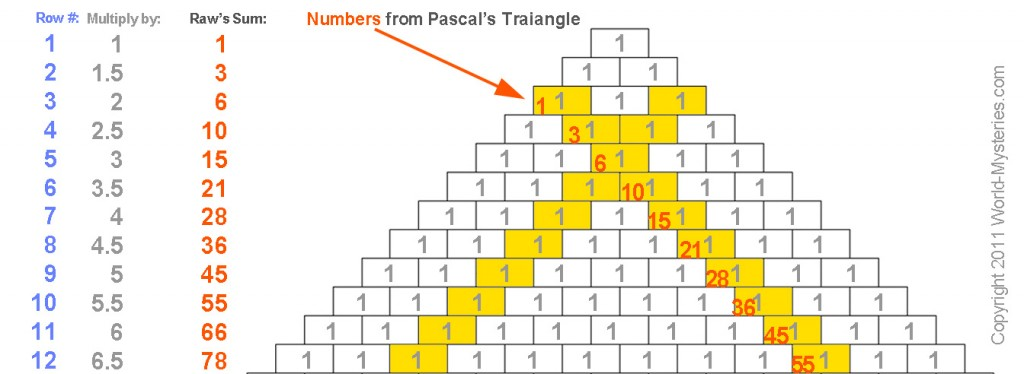Triangular Numbers

## The Fibonacci series has a pattern that repeats every 24 numbers

Numeric reduction is a technique used in analysis of numbers in which all the digits of a number are added together until only one digit remains.  As an example, the numeric reduction of 256 is 4 because 2+5+6=13 and 1+3=4.

Applying numeric reduction to the Fibonacci series produces an infinite series of 24 repeating digits:

1, 1, 2, 3, 5, 8, 4, 3, 7, 1, 8, 9, 8, 8, 7, 6, 4, 1, 5, 6, 2, 8, 1, 9

If you take the first 12 digits and add them to the second twelve digits and apply numeric reduction to the result, you find that they all have a value of 9.

 1st 12 numbers 1 1 2 3 5 8 4 3 7 1 8 9 2nd 12 numbers 8 8 7 6 4 1 5 6 2 8 1 9 Numeric reduction – Add rows 1 and 2 9 9 9 9 9 9 9 9 9 9 9 18 Final numeric reduction – Add digits of result 9 9 9 9 9 9 9 9 9 9 9 9

This pattern was contributed both by Joseph Turbeville and then again by a mathematician by the name of Jain.

The number is on the left, horizontal addition result is on the right. Notice how the 1,2,4,8,7,5 number pattern repeats indefinitely.

 1 = 1 2 = 2 4 = 4 8 = 8 16 = 7 32 = 5 64 = 1 128 = 2 256 = 4 512 = 8 1,024 = 7 2,048 = 5 4,096 = 1 8,192 = 2 16,384 = 4 32,768 = 8 65,536 = 7 131,072 = 5 262,144 = 1 524,288 = 2 1,048,576 = 4 2,097,152 = 8 4,194,304 = 7 8,388,608 = 5 16,777,216 = 1 33,554,432 = 2 67,108,864 = 4 134,217,728 = 8 268,435,456 = 7 536,870,912 = 5 1,073,741,824 = 1 2,147,483,648 = 2 4,294,967,296 = 4 8,589,934,592 = 8

At this point some of you might be thinking, “What in the world do these number patterns have to do with real world applications?” These number groupings piece together into a jig-saw-like puzzle pattern that perfectly demonstrates the way energy flows. Our base-ten decimal system is not man made, rather it is created by this flow of energy. Amazingly, after twenty years of working with this symbol and collaborating with engineers and scientists, Marko discovered that the 1,2,4,8,7,5 was a doubling circuit for a very efficient electrical coil. There was still one more very important number pattern to be realized. On the MATHEMATICAL FINGER PRINT OF GOD notice how the 3, 9, and 6 is in red and does not connect at the base. That is because it is a vector. The 1,2,4,8,7,5 is the third dimension while the oscillation between the 3 and 6 demonstrates the fourth dimension, which is the higher dimensional magnetic field of an electrical coil. The 3, 9, and 6 always occur together with the 9 as the control. In fact, the Yin/Yang is not a duality but rather a trinary. This is because the 3 and 6 represent each side of the Yin/Yang and the 9 is the “S” curve between them. Everything is based on thirds. We think that the universe is based on dualities because we see the effects not the cause.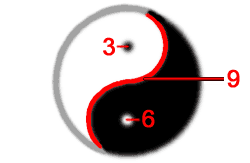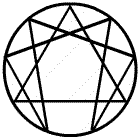### Titus-Bode Law and Pascal’s Triangle

In 1768, Bode published his popular book, “Anleitung zur Kenntnis des gestirnten Himmels” [Instruction for the Knowledge of the Starry Heavens]. In this book, he described an empirical law on planetary distances, originally found by J.D. Titius (1729-96), now called “Bode’s Law” or “Titius-Bode Law”.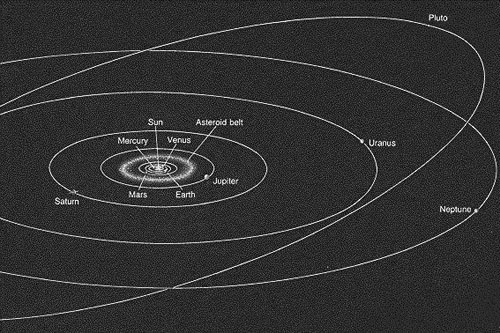The original formula was:

a = ( n + 4 ) / 10
where n=0,  3,  6,  12,  24,  48,  96,  192, 384, …

To find the mean distances of the planets, begin with the following simple sequence of numbers:
0     3     6     12     24     48     96     192     384   …
These numbers (after 0) derive from the Pascal’s triangle as the sum of a row added to the sum of the previous row:

Row 1:  2+1=3
Row 2:  4+2=6
Row 3:  8+4=12
Row 4:  16+8=24
Row 5:  32+16=48
Row 6:  64+32=96
Row 7:  128+64=192
Row 8:  256+128=384

Next, add 4 to each number and you will get:
4     7     10     16     28     52     100     196     388
Then divide it by 10:
0.4     0.7     1.0     1.6     2.8     5.2     10.0     19.6     38.8

The resulting sequence is very close to the distribution of mean distances of the planets from the Sun
expressed in Astronomical Units (AU):

 Body Actual distance (A.U.) Bode’s Law Mercury 0.39 0.4 Venus 0.72 0.7 Earth 1.00 1.0 Mars 1.52 1.6 asteroid belt 2.77 2.8 Jupiter 5.20 5.2 Saturn 9.54 10.0 Uranus 19.19 19.6 Neptune 30.06 n/a Pluto 39.44 38.8

1 AU is approximately the mean Earth–Sun distance equal AU = 149.597 *106 km

All planets (and asteroid belt) fit Titus-Bode Law except for Neptune!

Here is another way to connect Bode’s Law with Pascal’s Triangle
(Source: http://milan.milanovic.org/math/english/titius/titius.html)

 Planet k Pascal Triangle bin(k) Mercury 1 1 0 Venus 2 1 + 1 1 Earth 3 1 + 2 + 1 2 Mars 4 1 + 3 + 3 + 1 4 Planet V 5 1 + 4 + 6 + 4 + 1 8 Jupiter 6 1+ 5+10+ 10 + 5 + 1 16 Saturn 7 1+6 +15+20+ 15 + 6 + 1 32 Uranus 8 1+7+21+35+35+ 21 + 7 + 1 64 Neptune 9 bin(7) + bin(8) 96 Pluto 9 1+8+28+56+70+56+ 28 + 8 + 1 128

The modern formulation of the Titus-Bode law is that the mean distance a of the planet from the Sun in astronomical units ( AU = 149.597 *106 km ) is:

a = 0.4 + 0.3 x k

where ”k’=0,1,2,4,8,16,32,64,128 (sequence of powers of two – from Pascal’s Triangle)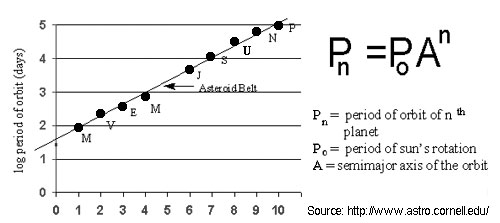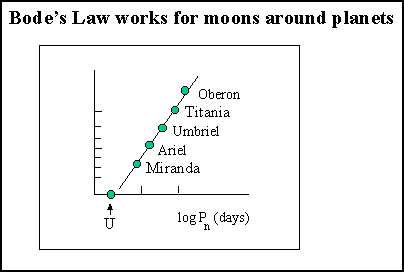Image Source (2 images above): http://www.astro.cornell.edu/

## PS1: Spira Solaris

A quotation from Ovid provided by Nicole Oresme in his major work, Le livre du ciel et du monde:

“The three-fold number is present in all things whatsoever; nor did we ourselves discover this number, but rather nature teaches it to us”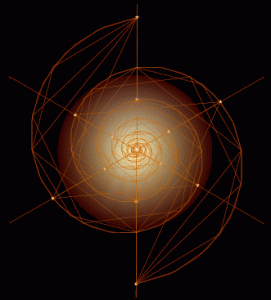Image Source: http://www.spirasolaris.ca/sbb4d2.html

Subject Related:

## PS2: “Pi”

Pi = 3.141 592 653 589 793 238 462 643 383 279 502 884 197 169 399 375…

Visitor’s comment submitted on Dec 9, 2011  by “Megabites4u”

I have always been interested in astronomy and cosmology from the view point of ancient cultures, but that aside, one has to look at their overall understanding of calendars, time and dates, buildings, sacred monuments, mathematics, geometry, their city centers, trade routes, technology, language of priests and peasants, the language of their Gods and the way they civilized the population, war and kingship, battles and war tools, what they taught, their laws, traditions, dialects oral or written, influences that affect stability, artifacts and materials used, whom they went to for guidance or intervention, medicine and how they cultivated or divided the land, their desire or aspirations, skills of carpenters, masons, miners, access to water and spices used, establishment of trade routes, names of famous writes or advisers and leaders that supported the structure and community and how they protected themselves from outsiders etc, etc.

There are so many theories being put forward by G Hancock, R Buval and others and i am in no doubt that we have to come to the “table” with an open mind. We know that humans have existed for 100 million years and can draw the broken tree of our ancestors. We need to explain how different parts of Earth developed as it is clear that whilst the middle-east was in the bronze age, other parts were still existing in the stone age (3rd world status), but they may have supplied materials for bronze implements, without having the technology to make such products. The ice age may not have covered Europe with a ice sheet 2km thick, maybe some pockets remained where people lived in isolation, we do not know all the facts. We cannot trust GOVTs or scientists to tell us everything – they are not idiots. Look at the mess we are in now and they will deny all knowledge that they had some notion of the suffering – we are groomed not to tell the truth, because if we get away with it, that is all to our advantage for survival.

I do believe that each of us has to look at all the aspects as far back as possible, and see how the facts need to be interpreted for all humanity. This is our ancient heritage, whether this relates to Pyramids or Stone Circles, any artifact has to be placed as to identify what was the thinking process and what was its purpose. If we are divided in opinion we will fail to get to the truth.

Give you an example: we know that even nomadic hunters had to follow the food trail and moved from place to place in order to survive. Once we started living in a fixed point, we had to get the food, water, tools, knowledge etc to come to us. We had to therefore interact, so whom did we interact with and why? Being fixed meant suffering bad and good conditions, so how did we survive the difficult months, how did we adopt to changing climate and natural disasters, disease and in fighting.

When did we first seem to know about the mechanics of the Earth, by looking at the stars and building an idea of time, by looking at the day/night cycles, months and seasons, so we knew when to sew and harvest, when to have children to give the best chance of survival, how to explain these movements of stars to new generations and give them a better chance of survival. When did we look at each aspect of life as a cycle, like the moon and planets nearest to our own Earth. How did we encode this information of cycles of time and space into great Epic Sagas developed into cosmic cycles and myths and legends so each generation could remember their heritage and understand social responsibility.

We talk of Plato and Atlantis, King Solomon’s mines, the Temple of Solomon, The Books of the Jews and Israelites and how the cosmos is 13.6999xxxxx billion years old, but despite all this know how and intellectual depth we have more problems today then our ancestors faced, more financial tsunamis each day and maybe its just my opinion but we have not found ONE DAY on Earth we celebrate peace, it eludes us, its not in our grasp, but we are more interested in wealth and achieving immortality. If the fact that we could achieve IMMORTALITY just to experience being inside every atom at the same time, and this was FREE, would the GOVT tell you or the priests tell you? Absolutely NOT, because they will not be able to have control over you, but it is a state of happiness you can get by searching in the right places and not just for yourself but for all humanity, so if you achieved it yourself, would you share it? Absolutely NOT, because people will treat you, as if you are mad.

We know that life is hidden inside a millet seed, so IMMORTALITY is also hidden inside a millet seed, would be a good way of looking, would you agree?

In all of humanity I know only TWO people who have IMMORTALITY, one died and lived again and the other never had experience of death, he never died and yet in all our search we do not understand one and the other is never mentioned, in fact he is only mentioned once of in Genesis and so you have to ask why these two, what was special about these two and how do all the other facts try to help us to achieve the same degree of wealth and understanding they had to become like them.

Let me leave you with this fact for your path to understanding:

1 = God
0 = Contains God (nothingness still has the presence of God)
8 = infinity or you can call it 10 to the power of infinity, so it contains everything.

4 x 27 = 108
108/5 = 21.6

3,6,12,24,27,36,54,60,72,108,144,180,216,252,288,324,360,540,720, 1296, 2592,4320, 5184 etc are TIME SPACE constants and hidden inside in PI = 3.141592654

NOW LOOK:

3 x 1 = 3
3 x 1 x 4 = 12
3 x 1 x 4 x 1 x 5 = 60
3 x 1 x 4 x 1 x 5 x 9 = 540
3 x 1 x 4 x 1 x 5 x 9 x 2 = 1080
3 x 1 x 4 x 1 x 5 x 9 x 2 x 6 = 6480
3 x 1 x 4 x 1 x 5 x 9 x 2 x 6 x 5 = 32400
3 x 1 x 4 x 1 x 5 x 9 x 2 x 6 x 5 x 4 = 129600

SEE I TOLD YOU – Its all in the heart of a millet seed.

The Sumerians, Akkadians, Babylonians, Egyptians, Greeks, Romans and later Muslim scholars and the Western World adopted all these value and universal constants and we are just not sure how to use these to understand Divine Light and therefore achieve our goal. It seems we still are looking for Aliens and Atlantis – digging here and there and yet it is right in front of us, if we could only see!

GOOD LUCK and hope that one day we all live in peace and unity, because someone is trying hard for us NOT to achieve this and has so far succeeded for 10500 years!

Bye 4 Now – xxxxxx

### Note: Here are first 105  digits of “Pi”:

3,1,4,1,5,9,2,6,5,3,5,8,9,7,9,3,2,3,8,4,6,2,6,4,3,3,8,3,2,7,9,5,0,2,8,8,4,1,9,7,1,6,9,3,9,9,3,7,5,
1,0,5,8,2,0,9,7,4,9,4,4,5,9,2,3,0,7,8,1,6,4,0,6,2,8,6,2,0,8,9,9,8,6,2,8,0,3,4,8,2,5,3,4,2,1,1,7,0,
6,7,9,8,2,1,4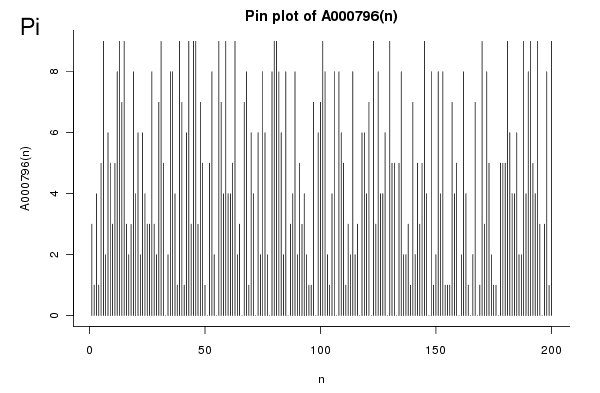Pi Plot – Source: http://oeis.org/A000796

f we approximate Pi as 3.141592653…

3 x 1 = 3
3 x 1 x 4 = 12
3 x 1 x 4 x 1 x 5 = 60
3 x 1 x 4 x 1 x 5 x 9 = 540  :::  540/360=1.5
3 x 1 x 4 x 1 x 5 x 9 x 2 = 1080  :::  1080/360=3
3 x 1 x 4 x 1 x 5 x 9 x 2 x 6 = 6480  :::  6480/360=18
3 x 1 x 4 x 1 x 5 x 9 x 2 x 6 x 5 = 32400  :::  32400/360=90
3 x 1 x 4 x 1 x 5 x 9 x 2 x 6 x 5 x 3 = 97200  :::  97200/360=270

## PS3: Phi

### Phi = 1.61803 39887 49894 84820 45868 34365 63811 77203 09179 80576

Phi, 1.618 … ,  the Golden Ratio in life and the universe.

Phi + 1 = Phi 2

(6/5 ) x  Phi 2 = 3.1416, which approximates Pi.

The dimensions of the Earth and Moon are in Phi relationship, forming a Golden Triangle:### Relative planetary distances average to Phi

The average of the mean orbital distances of each successive planet in relation to the one before it approximates phi:

 Planet Mean distance in million kilometers per NASA Relative mean distance where Mercury=1 Mercury 57.91 1.00000 Venus 108.21 1.86859 Earth 149.60 1.38250 Mars 227.92 1.52353 Ceres 413.79 1.81552 Jupiter 778.57 1.88154 Saturn 1,433.53 1.84123 Uranus 2,872.46 2.00377 Neptune 4,495.06 1.56488 Pluto 5,869.66 1.30580 Total 16.18736 Average 1.61874 Phi 1.61803 Degree of variance (0.00043)

### Phi appears in:

PS 3 Source:  http://www.goldennumber.net

## PS4: Number 666

Note: This segment source: http://www.symbolicliving.com/forum/forum.php

108 x 2= 216 = 6 x 6 x 6 , 666 is the number of the Sun (and also number of the Beast)
108  has 12 divisors:   1, 2, 3, 4, 6, 9, 12, 18, 27, 36, 54, 108

### Some meanings of the number 666

The number 666 is number of the Sun.
Why number 666?

Just few small examples:

Sentence from the Bible: YHVH Shemesh or “The Lord is the Sun”.
YHVH = yod + heh + vau + he = 10 + 5 + 6 + 5 = 26
Shemesh = shin + mem + shin = 300 + 40 + 300 = 640
26 + 640 = 666

The number 666 has 12 divisors {1, 2, 3, 6, 9, 18, 37, 74, 111, 222, 333, 666}.

Sun (in mythologies: Christ, Buddha, Balder, Horus, Bacchus, Dyonisos, Attis, Mithra,…) has 12 disciples, or 12 signs of zodiac.
Sun passes through 12 constellations on the ecliptic belt.

“I am he; I am the first, I also am the last.” Numerical value of the paragraph is 216 or 6 x 6 x 6.
Here God proclaims that he is the Sun.

Hebrew word “Aryeh” or “lion”:
Aryeh = aleph + resh + yod + heh = 1 + 200 + 10 + 5 = 216 or 6 x 6 x 6. The lion in all mythologies and all religions symbolizes the Sun, the true “god” of the solar system.
Hebrew word “Gehvurah” for might, strength:
Gehvurah = gimel + beth + vau + resh + heh = 3 + 2 + 6 + 200 + 5 = 216 or 6 x 6 x 6.
If two words or sentences have the same numerical value, they have similar meaning like:
Aryeh = 216 –> lion
Gevurah = 216 –> strength
Therefore, lion is strong, and so is the Sun

“The heaven is my throne, and the earth is my footstool” (Is 66.1):
Numerical value = 216 or 6 x 6 x 6. The heaven is my throne = the sky is the throne of Sun. The earth is my footstool = solar rays influence all life on Earth.
“He hath made the earth by his power, he hath established the world by his wisdom, and hath stretched out the heavens by his discretion.”:
He hath made the earth or “Asah Aretz”= ayin + shin + heh + aleph + resh + tzaddi = 70 + 300 + 5 + 1 + 200 + 90 = 666

“God the LORD, he that created the heavens”:
“HaEl YHVH Bo-rah Shamayim” = 666

“He that spread forth the earth”:
“Raqa Aretz” = 666

“Let there be lights”:
“Yahi Moroth” = 666

“Like God”:
“K’Elohim” = 666. The Sun is metaphorically speaking like a God in religion.

“The Holy Crown”:
“Nezar HaQadosh” = 666. This is the real meaning of the crown of thorns. It refers to actual solar rays which seem like a crown or halo on the Sun (usually depicted around head of Jesus).

“From God”:
On Greek “Para Theou” = pi + alpha + rho + alpha + thetha + epsilon + omicron + upsilon = 80 + 1 + 100 + 1 + 9 + 5 + 70 + 400 = 666

“Now the weight of gold that came to Solomon in one year was six hundred threescore and six talents of gold.”
Threescore means 60. “Six hundred threescore and six talents of gold” equals 666 talents of gold. In alchemical symbolism, gold represents Sun.

These are just a few examples of solar attributes of the number 666.

Perfect cube numbers:
1, 8, 27, 64, 125, 216, 343, 512, 729, 1000, 1331, 1728
The 6th number is 216 or 6 x 6 x 6 and 6th sphere on the Tree of Life is Sun or Tiphareth on Hebrew.
37th triangular number is 666. 3 + 7 = 10 or tetraktys, Tree of Life.

Number 666 in binary is 1010011010, which contains 10 digits, or first ten Ideas in Universal Mind, the Tree of Life.

In magickal square (kamea) of the Sun, all numbers are added in any direction to produce the sum of 111, and since there is 6 columns and lines, 111 x 6 = 666.

In 1 Kings 10:14, Old Testament, there is a story of King Solomon receiving 666 coins of gold.
Sol-Om-On is the name of Sun in three languages: Word SOL means “Sun” in Latin, OM or AUM means “Sun” in Hindu Sanskrit and ON means “Sun” in Egyptian.

If we add up all numbers from 1-36 it will result in 666. 36 is sacred Pythagorean number of Sun.
King Solomon’s seal is 6-pointed star, the hexagram.
So, again the number of the Sun is 6 or 666.

Carbon (CO2) which is the chemical basis of all physical life on Earth has 6 protons, 6 neutrons and 6 electrons. The shape of carbon molecules points to hexagram, the symbol of unity.

The perfect cube (6 X 6 X 6) is perfect ashlar of Freemasonry and Philosophers Stone.
Number 216 or 6 x 6 x 6 is also produced of 3 x 3 x 3 + 4 x 4 x 4 + 5 x 5 x 5, the cubical number of perfect Pythagorean triangle c2 = a2 + b2. These ratios of 3:4:5 is ancient Egyptian symbol for Trinity of Osiris, Isis and Horus.

Serpent (TITh)= 419
Lion (ARIH)= 216
+ 31 (AL – the God associated to Chesed and the Key to Crowley’s Liber AL) = 666. Trinity of Serpent (female-energy), Lion (male-movement) and Son (neutral-individual life).

Interesting, multiples of 3 multiplied by 37 = repeating digits

(1×3) or 1+1+1=3 x 37 = 111
(2×3) or 2+2+2=6 x 37 = 222
(3×3) or 3+3+3=9 x 37 = 333
(4×3) or 4+4+4=12 x 37 = 444
(5×3) or 5+5+5=15 x 37 = 555
(6×3) or 6+6+6=18 x 37 = 666
(7×3) or 7+7+7=21 x 37 = 777
(8×3) or 8+8+8=24 x 37 = 888
(9×3) or 9+9+9=27 x 37 = 999

Divisors:

108 has 12 divisors:  1, 2, 3, 4, 6, 9, 12, 18, 27, 36, 54, 108
216 has 16 divisors:  1, 2, 3, 4, 6, 8, 9, 12, 18, 24, 27, 36, 54, 72, 108, 216
360 has 24 divisors:  1, 2, 3, 4, 5, 6, 8, 9, 10, 12, 15, 18, 20, 24, 30, 36, 40, 45, 60, 72, 90, 120, 180, 360
666 has 12 divisors:  1, 2, 3, 6, 9, 18, 37, 74, 111, 222, 333, 666